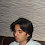## Friday, March 11, 2011

### Plotting Indifference Curves with R Contour Function

The following post at Constructing Difference Curves - Part 3 from economics.about.com provides a discussion on indifference curves (but actually I think they are isoquants) and how to construct them. I think I have a grasp on how to do this in R if you define the utility function as z =  √(x*y) . For the x and y data I used modified data from the article mentioned above, and then plotted some simulated data.

The data I used for x and y (which I modified-see documentation in the R-code) is listed below:

x: 1,2,3,4,5,6,7,8
y: 10,10,10,15,15,30,60,90

The R code below reads in the data and plots level sets or indifference curves for both the data above and the simulated data. This very basic, for full documentation ( and options for x,y limits, levels etc.) of the contour function in R see here.

Edit: for the second part of the program, if I switch the values of x and y I get indifference curves that are convex to the origin as they should be- the correction can be also represented much more compactly as:

```contour(
xyT<-t(as.matrix(cbind(x<-seq(0,20,by=2),y<-seq(0,100,by=10)))),
z<-as.matrix(sqrt(x*y))
)```

Created by Pretty R at inside-R.org

See program below:

```# *------------------------------------------------------------------
# | PROGRAM NAME: INDIFFERENCE_CURVES_R
# | DATE: 3/11/11
# | CREATED BY:  MATT BOGARD
# | PROJECT FILE:  P:\R  Code References
# *----------------------------------------------------------------
# | PURPOSE: MORE TO PLOT LEVEL SETS USING THE CONTOUR FUNCTION THAN
# |          TO ACTUALLY PLOT INDIFFERENCE CURVES
# *------------------------------------------------------------------
# |
# |  1: references: http://stat.ethz.ch/R-manual/R-devel/library/graphics/html/contour.html
# |  2:
# |  3:
# |*------------------------------------------------------------------
# |
# |
# |*------------------------------------------------------------------
# | CONTENTS:
# |
# |  PART 1: explicit data
# |  PART 2: simulated data  (sort of) - not really indifference curves
# |  PART 3:
# *-----------------------------------------------------------------
# |
# |
# *------------------------------------------------------------------

# *------------------------------------------------------------------
# | PART 1:   explicit data
# *-----------------------------------------------------------------

# raw data

x <- c(1,2,3,4,5,6,7,8)
y <- c(10,10,10,15,15,30,60,90)

# note- for the contour function to work below, x and y
# These must be in ascending order-based on the contour documentation
#  which is the opposite of how the data was presented at

# put x and y in a matrix

xy <- as.matrix(cbind(x,y))

# transpose xy

xyT <- t(xy)

# define function z as a matrix

z <- as.matrix(sqrt(x*y))

# plot the countour plot / specified level sets

contour(xyT,z) # all levels

contour(xyT,z, levels =c(10,20,50)) # specified levels for z

# *------------------------------------------------------------------
# | PART 2:   simulated data  (sort of) - not really indifference curves
# *-----------------------------------------------------------------

rm(list=ls()) # get rid of any existing data

ls() # display active data -should be null

# define x and y

x <- seq(0,100,by=10)
y <- seq(0,20,by=2)

# put x and y in a matrix

xy <- as.matrix(cbind(x,y))

# transpose xy

xyT <- t(xy)

# define function z as a matrix

z <- as.matrix(sqrt(x*y))

contour(xyT,z)```
Created by Pretty R at inside-R.org

1.how can I graph a function as a level curve?

2.It is my understanding that all of the examples above are level curves. For instance, the second part of the program plots all of the level curves for the function y = square root(x*y). I would refer to the referenced R documentation for more details.

3.I need to plot as a level curves -but I do not know how?- the next function:

z=100*(x-15)^2 +20*(28-x)^2+100(y-x)^2+20*(38-x-y)^2

how can I do it ?

4.I need to plot three indifference curve for the level 100, 200, 300
my utility function is u(x,y)=3x^2+2y
how can i do it?